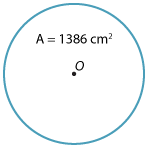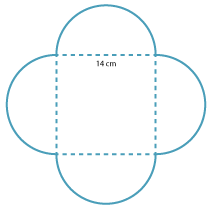### The formula for area of a circle

The dissections shown above strongly suggest that the correct formula for the area of a circle with radius r is

A = πr2

A formal proof of this result requires an understanding of the concept of limits, which will be studied in senior mathematics.

#### Example 1

1. Find the area of a circle whose radius is 7 cm.
2. Find the area of a circle whose diameter is 7 cm.

1. in terms of π
2. as an approximate value, using $$\pi \approx \dfrac{22}{7}.$$

#### Solution

\begin{align}{\bf \text{a i}}\hspace{22px} A &= \pi r^2\\\\ &= 49\pi\ \text{cm}^2\end{align} \begin{align}{\bf \text{a ii}}\hspace{18px} A &= 49\pi\\\\ &\approx 49 × \dfrac{22}{7}\\\\ &= 154\ \text{cm}^2\end{align}

b i Since the diameter is 7 cm, the radius is $$\dfrac{7}{2}\ \text{cm}$$

\begin{align}A &= \pi r^2\\\\ &= \dfrac{49}{4}\pi\ \text{cm}^2\end{align}
\begin{align}{\bf \text{b ii}}\hspace{18px} A &=\dfrac{49}{4}\pi\\\\ &\approx \dfrac{49}{4} × \dfrac{22}{7}\\\\ &=38\dfrac{1}{2}\ \text{cm}^2\end{align}

#### Example 2

A circle has area $$1386\ \text{cm}^2.$$ Using $$\pi \approx\dfrac{22}{7},$$ find the approximate value of the radius.#### Solution

\begin{align}A &= \pi r^2\\\\ \pi r^2 &= 1386\\\\ r^2 &= \dfrac{1386}{\pi}\\\\ &\approx 1386 ÷\dfrac{22}{7} \\\\ &\approx \dfrac{1386}{1}× \dfrac{7}{22} \\\\ &= 441\\\\ r &= \sqrt{441}\\\\ &= 21\ \text{cm}\end{align}

#### Example 3Four semicircles are drawn along the edge of a square with side length 14 cm.

1. Find the area of the entire region, giving your answer in terms of π.
2. Find the approximate value of the area, using $$\pi \approx \dfrac{22}{7}$$ .

#### Solution

1. Area region = area of the square + 2 × area of the circle
\begin{align}\text{Area square} &= 14 × 14\\\\ &= 196\ \text{cm}^2\end{align} \begin{align}\text{Radius of circle} &= 7\ \text{cm}\end{align} \begin{align}\text{Area of circles} &= 2 × \pi × 7^2\\\\ &= 2 × \pi × 49\\\\ &= 98\pi\ \text{cm}^2\end{align} Hence the area of the region = (196 + 98π) cm$$^2$$
2. \begin{align}\text{Area} &≈ 196 + 98 × \dfrac{22}{7}\\\\ &= 196 + 308\\\\ &= 504\ \text{cm}^2\end{align}